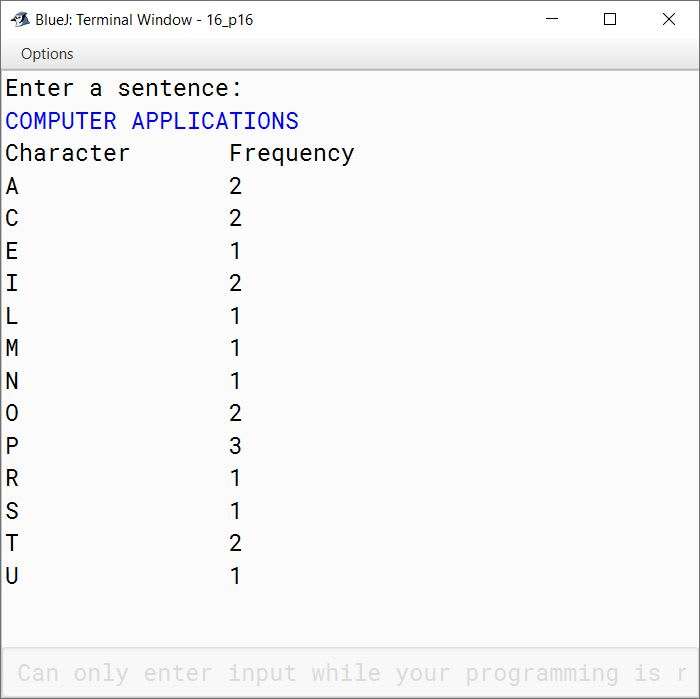# Solved 2010 Question Paper ICSE Class 10 Computer Applications

## Section A

#### Question 1

(a) Define the term Bytecode.

Java compiler converts Java source code into an intermediate binary code called Bytecode. Bytecode can't be executed directly on the processor. It needs to be converted into Machine Code first.

(b) What do you understand by type conversion? How is implicit conversion different from explicit conversion?

The process of converting one predefined type into another is called type conversion. In an implicit conversion, the result of a mixed mode expression is obtained in the higher most data type of the variables without any intervention by the user. For example:

```int a = 10;
float b = 25.5f, c;
c = a + b;```

In case of explicit type conversion, the data gets converted to a type as specified by the programmer. For example:

```int a = 10;
double b = 25.5;
float c = (float)(a + b);```

(c) Name two jump statements and their use.

break statement, it is used to jump out of a switch statement or a loop. continue statement, it is used to skip the current iteration of the loop and start the next iteration.

(d) What is Exception? Name two exception handling blocks.

An exception is an event, which occurs during the execution of a program, that disrupts the normal flow of the program's instructions. Two exception handling blocks are try and catch.

(e) Write two advantages of using functions in a program.

1. Methods help to manage the complexity of the program by dividing a bigger complex task into smaller, easily understood tasks.
2. Methods help with code reusability.

#### Question 2

(a) State the purpose and return data type of the following String functions:

1. indexOf()
2. compareTo()

1. indexOf() returns the index within the string of the first occurrence of the specified character or -1 if the character is not present. Its return type is int.
2. compareTo() compares two strings lexicographically. Its return type is int.

(b) What is the result stored in x, after evaluating the following expression?

```int x = 5;
x = x++ * 2 + 3 * --x;```

x = x++ * 2 + 3 * --x
⇒ x = 5 * 2 + 3 * 5
⇒ x = 10 + 15
⇒ x = 25

(c) Differentiate between static and non-static data members.

Static Data MembersNon-Static Data Members
They are declared using keyword 'static'.They are declared without using keyword 'static'.
All objects of a class share the same copy of Static data members.Each object of the class gets its own copy of Non-Static data members.
They can be accessed using the class name or object.They can be accessed only through an object of the class.

(d) Write the difference between length and length().

lengthlength()
length is an attribute i.e. a data member of array.length() is a member method of String class.
It gives the length of an array i.e. the number of elements stored in an array.It gives the number of characters present in a string.

(e) Differentiate between private and protected visibility modifiers.

Private members are only accessible inside the class in which they are defined and they cannot be inherited by derived classes. Protected members are also only accessible inside the class in which they are defined but they can be inherited by derived classes.

#### Question 3

(a) What do you understand by the term data abstraction? Explain with an example.

Data Abstraction refers to the act of representing essential features without including the background details or explanations. A Switchboard is an example of Data Abstraction. It hides all the details of the circuitry and current flow and provides a very simple way to switch ON or OFF electrical appliances.

(b)(i) What will be the output of the following code?

```int m = 2;
int n = 15;
for(int i = 1; i < 5; i++);
m++;
--n;
System.out.println("m = " + m);
System.out.println("n = " + n);```

##### Output
``````m=6
n=14
``````
##### Explanation

As there are no curly braces after the for loop so only the statement `m++;` is inside the loop. Loop executes 4 times so `m` becomes 6. The next statement `--n;` is outside the loop so it is executed only once and `n` becomes 14.

(b)(ii) What will be the output of the following code?

```char x = 'A';
int m;
m = (x == 'a')? 'A' : 'a';
System.out.println("m = " + m);```

##### Output
``````m = 97
``````
##### Explanation

The condition x == 'a' is false as X has the value of 'A'. So, the ternary operator returns 'a' that is assigned to m. But m is an int variable not a char variable. So, through implicit conversion Java converts 'a' to its numeric ASCII value 97 and that gets assigned to m.

(c) Analyze the following program segment and determine how many times the loop will be executed and what will be the output of the program segment.

```int k = 1, i = 2;
while(++i < 6)
k *= i;
System.out.println(k);```

##### Output
``````60
``````
##### Explanation

This table shows the change in values of i and k as while loop iterates:

ikRemarks
21Initial values
331st Iteration
4122nd Iteration
5603rd Iteration
660Once i becomes 6, condition is false and loop stops iterating.

Notice that `System.out.println(k);` is not inside while loop. As there are no curly braces so only the statement `k *= i;` is inside the loop. The statement `System.out.println(k);` is outside the while loop, it is executed once and prints value of k which is 60 to the console.

(d) Give the prototype of a function check which receives a character ch and an integer n and returns true or false.

boolean check(char ch, int n)

(e) State two features of a constructor.

1. A constructor has the same name as that of the class.
2. A constructor has no return type, not even void.

(f) Write a statement each to perform the following task on a string:

1. Extract the second-last character of a word stored in the variable wd.
2. Check if the second character of a string str is in uppercase.

1. char ch = wd.charAt(wd.length() - 2);
2. boolean res = Character.isUpperCase(str.charAt(1));

(g) What will the following function return when executed?

1. Math.max(-17, -19)
2. Math.ceil(7.8)

1. -17
2. 8.0

(h)(i) Why is an object called an instance of a class?

A class can create objects of itself with different characteristics and common behaviour. So, we can say that an Object represents a specific state of the class. For these reasons, an Object is called an Instance of a Class.

(h)(ii) What is the use of the keyword import?

import keyword is used to import built-in and user-defined packages into our Java program.

## Section B

#### Question 4

Write a program to perform binary search on a list of integers given below, to search for an element input by the user. If it is found display the element along with its position, otherwise display the message "Search element not found".

5, 7, 9, 11, 15, 20, 30, 45, 89, 97

```import java.util.Scanner;

public class KboatBinarySearch
{
public static void main(String args[]) {

Scanner in = new Scanner(System.in);
int arr[] = {5, 7, 9, 11, 15, 20, 30, 45, 89, 97};

System.out.print("Enter number to search: ");
int n = in.nextInt();

int l = 0, h = arr.length - 1, index = -1;
while (l <= h) {
int m = (l + h) / 2;
if (arr[m] < n)
l = m + 1;
else if (arr[m] > n)
h = m - 1;
else {
index = m;
break;
}

}

if (index == -1) {
}
else {
System.out.println(n + " found at position " + index);
}
}
}```
##### Output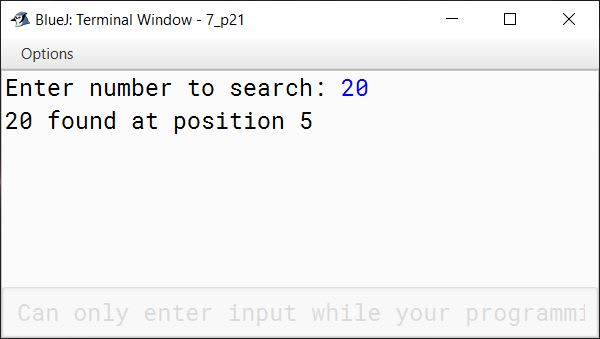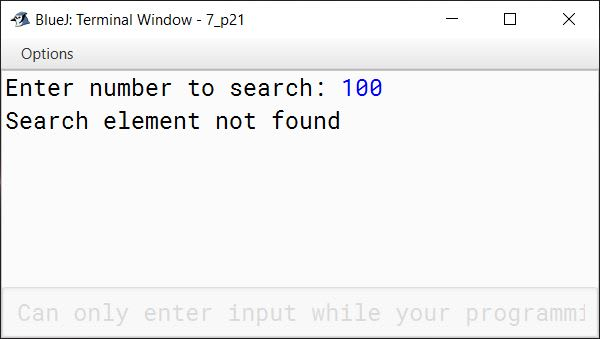#### Question 5

Define a class Student as given below:

Data members/instance variables:
name, age, m1, m2, m3 (marks in 3 subjects), maximum, average

Member methods:

1. A parameterized constructor to initialize the data members.
2. To accept the details of a student.
3. To compute the average and the maximum out of three marks.
4. To display the name, age, marks in three subjects, maximum and average.

Write a main method to create an object of a class and call the above member methods.

```import java.util.Scanner;

public class Student
{
private String name;
private int age;
private int m1;
private int m2;
private int m3;
private int maximum;
private double average;

public Student(String n, int a, int s1,
int s2, int s3) {
name = n;
age = a;
m1 = s1;
m2 = s2;
m3 = s3;
}

public Student() {
name = "";
age = 0;
m1 = 0;
m2 = 0;
m3 = 0;
maximum = 0;
average = 0;
}

public void accept() {
Scanner in = new Scanner(System.in);
System.out.print("Enter name: ");
name = in.nextLine();
System.out.print("Enter age: ");
age = in.nextInt();
System.out.print("Enter Subject 1 Marks: ");
m1 = in.nextInt();
System.out.print("Enter Subject 2 Marks: ");
m2 = in.nextInt();
System.out.print("Enter Subject 3 Marks: ");
m3 = in.nextInt();
}

public void compute() {
if (m1 > m2 && m1 > m3)
maximum = m1;
else if (m2 > m1 && m2 > m3)
maximum = m2;
else
maximum = m3;

average = (m1 + m2 + m3) / 3.0;
}

public void display() {
System.out.println("Name: " + name);
System.out.println("Age: " + age);
System.out.println("Subject 1 Marks: " + m1);
System.out.println("Subject 2 Marks: " + m2);
System.out.println("Subject 3 Marks: " + m3);
System.out.println("Maximum Marks: " + maximum);
System.out.println("Average Marks: " + average);
}

public static void main(String args[]) {
Student obj = new Student();
obj.accept();
obj.compute();
obj.display();
}
}```
##### Output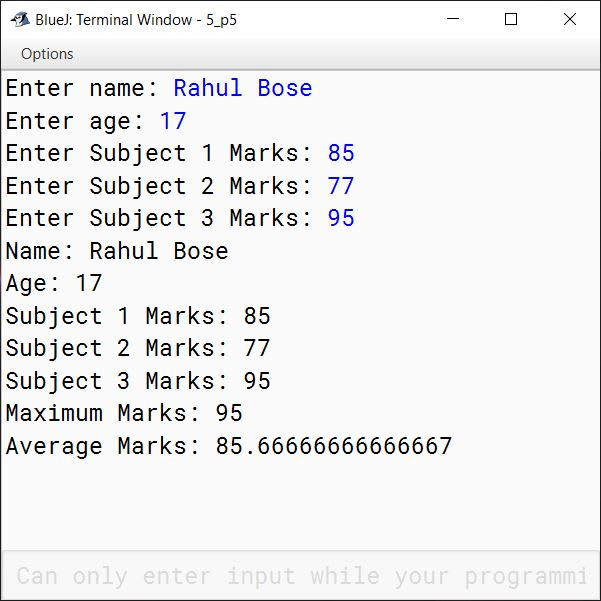#### Question 6

Shasha Travels Pvt. Ltd. gives the following discount to its customers:

Ticket AmountDiscount
Above Rs. 7000018%
Rs. 55001 to Rs. 7000016%
Rs. 35001 to Rs. 5500012%
Rs. 25001 to Rs. 3500010%
Less than Rs. 250012%

Write a program to input the name and ticket amount for the customer and calculate the discount amount and net amount to be paid. Display the output in the following format for each customer:

``````Sl. No.     Name    Ticket Charges     Discount      Net Amount
``````

(Assume that there are 15 customers, first customer is given the serial no (SI. No.) 1, next customer 2 …….. and so on)

```import java.util.Scanner;

public class KboatShashaTravels
{
public static void main(String args[]) {
Scanner in = new Scanner(System.in);
String names[] = new String;
int amounts[] = new int;

for (int i = 0; i < 15; i++) {
System.out.print("Enter " + "Customer " + (i+1) + " Name: ");
names[i] = in.nextLine();
System.out.print("Enter " + "Customer " + (i+1) + " Ticket Charges: ");
amounts[i] = in.nextInt();
in.nextLine();
}

System.out.println("Sl. No.\tName\t\tTicket Charges\tDiscount\t\tNet Amount");

for (int i = 0; i < 15; i++) {
int dp = 0;
int amt = amounts[i];
if (amt > 70000)
dp = 18;
else if (amt >= 55001)
dp = 16;
else if (amt >= 35001)
dp = 12;
else if (amt >= 25001)
dp = 10;
else
dp = 2;

double disc = amt * dp / 100.0;
double net = amt - disc;

System.out.println((i+1) + "\t" + names[i]
+ "\t" + amounts[i] + "\t\t"
+ disc + "\t\t" + net);
}
}
}```
##### Output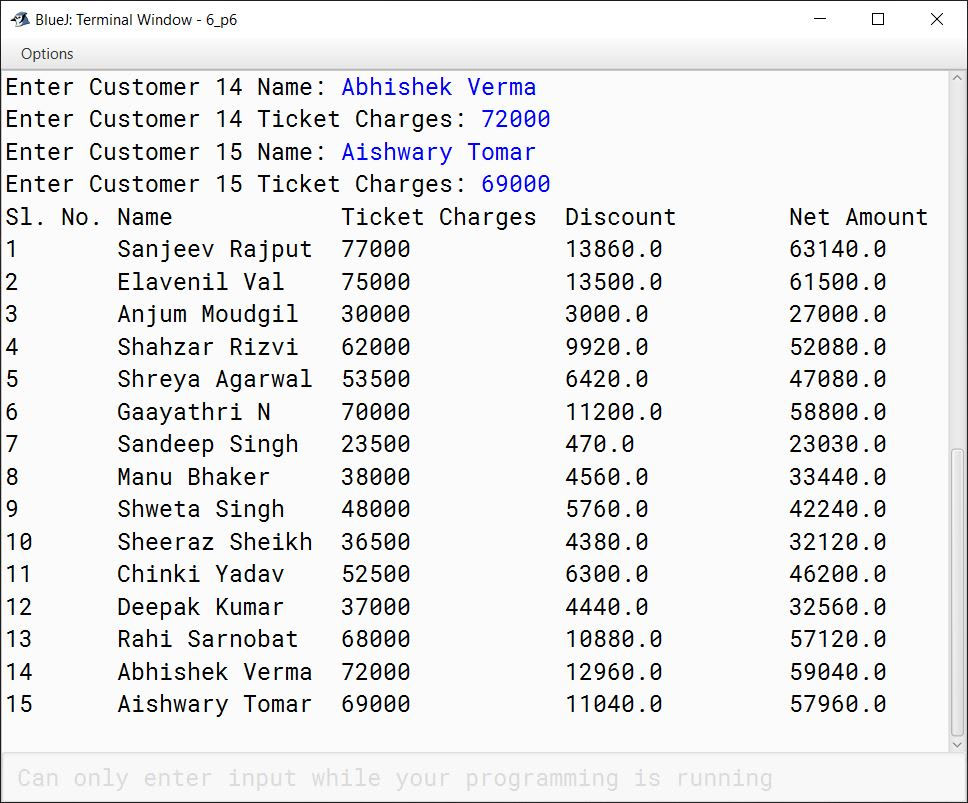#### Question 7

Write a menu driven program to accept a number from the user and check whether it is a Prime number or an Automorphic number.

(a) Prime number: (A number is said to be prime, if it is only divisible by 1 and itself)

Example: 3,5,7,11

(b) Automorphic number: (Automorphic number is the number which is contained in the last digit(s) of its square.)

Example: 25 is an Automorphic number as its square is 625 and 25 is present as the last two digits.

```import java.util.Scanner;

public class KboatPrimeAutomorphic
{
public static void main(String args[]) {
Scanner in = new Scanner(System.in);
System.out.println("1. Prime number");
System.out.println("2. Automorphic number");
int choice = in.nextInt();
System.out.print("Enter number: ");
int num = in.nextInt();

switch (choice) {
case 1:
int c = 0;
for (int i = 1; i <= num; i++) {
if (num % i == 0) {
c++;
}
}
if (c == 2)
System.out.println(num + " is Prime");
else
System.out.println(num + " is not Prime");
break;

case 2:
int numCopy = num;
int sq = num * num;
int d = 0;

/*
* Count the number of
* digits in num
*/
while(num > 0) {
d++;
num /= 10;
}

/*
* Extract the last d digits
* from square of num
*/
int ld = (int)(sq % Math.pow(10, d));

if (ld == numCopy)
System.out.println(numCopy + " is automorphic");
else
System.out.println(numCopy + " is not automorphic");
break;

default:
System.out.println("Incorrect Choice");
break;
}
}
}```
##### Output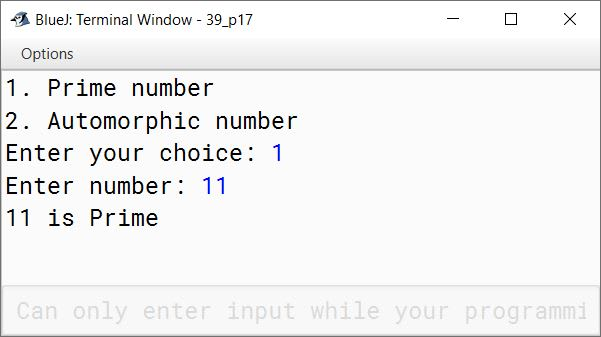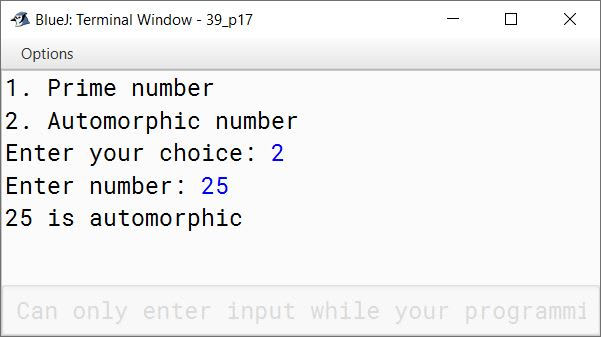#### Question 8

Write a program to store 6 elements in an array P and 4 elements in an array Q. Now, produce a third array R, containing all the elements of array P and Q. Display the resultant array.

InputInputOutput
P[ ]Q[ ]R[ ]
4194
6236
171
282
3 3
10 10
19
23
7
8

```import java.util.Scanner;

public class Kboat3Arrays
{
public static void main(String args[]) {

Scanner in = new Scanner(System.in);

int P[] = new int;
int Q[] = new int;
int R[] = new int;
int i = 0;

System.out.println("Enter 6 elements of array P:");
for (i = 0; i < P.length; i++) {
P[i] = in.nextInt();
}

System.out.println("Enter 4 elements of array Q:");
for (i = 0; i < Q.length; i++) {
Q[i] = in.nextInt();
}

i = 0;
while(i < P.length) {
R[i] = P[i];
i++;
}

int j = 0;
while(j < Q.length) {
R[i++] = Q[j++];
}

System.out.println("Elements of Array R:");
for (i = 0; i < R.length; i++) {
System.out.print(R[i] + " ");
}
}
}```
##### Output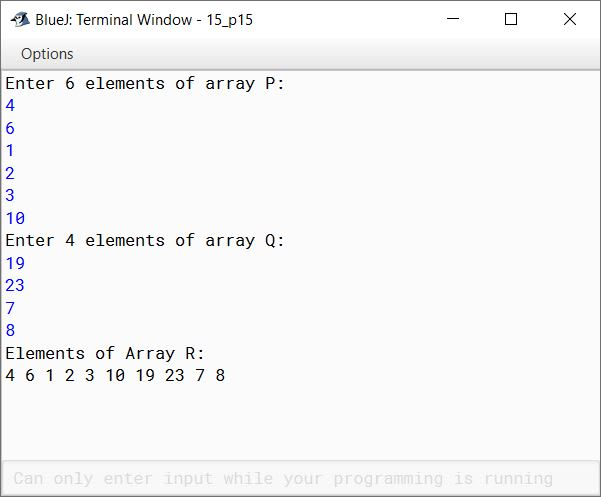#### Question 9

Write a program to input a sentence. Count and display the frequency of each letter of the sentence in alphabetical order.
Sample Input: COMPUTER APPLICATIONS
Sample Output:

CharacterFrequencyCharacterFrequency
A2O2
C2P3
I1R1
L2S1
M1T2
N1U1

```import java.util.Scanner;

public class KboatLetterFreq
{
public static void main(String args[]) {
Scanner in = new Scanner(System.in);
System.out.println("Enter a sentence:");
String str = in.nextLine();
str = str.toUpperCase();
int freqMap[] = new int;
int len = str.length();

for (int i = 0; i < len; i++) {
char ch = str.charAt(i);
if (Character.isLetter(ch)) {
int chIdx = ch - 65;
freqMap[chIdx]++;
}
}

System.out.println("Character\tFrequency");
for (int i = 0; i < freqMap.length; i++) {
if (freqMap[i] > 0) {
System.out.println((char)(i + 65)
+ "\t\t" + freqMap[i]);
}
}
}
}```
##### Output